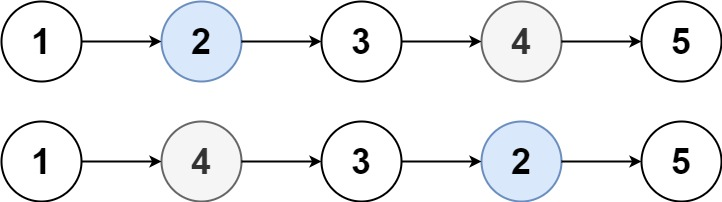Formatted question description: https://leetcode.ca/all/1721.html

# 1721. Swapping Nodes in a Linked List

Medium

## Description

You are given the head of a linked list, and an integer k.

Return the head of the linked list after swapping the values of the k-th node from the beginning and the k-th node from the end (the list is 1-indexed).

Example 1:Input: head = [1,2,3,4,5], k = 2

Output: [1,4,3,2,5]

Example 2:

Input: head = [7,9,6,6,7,8,3,0,9,5], k = 5

Output: [7,9,6,6,8,7,3,0,9,5]

Example 3:

Input: head = , k = 1

Output: 

Example 4:

Input: head = [1,2], k = 1

Output: [2,1]

Example 5:

Input: head = [1,2,3], k = 2

Output: [1,2,3]

Constraints:

• The number of nodes in the list is n.
• 1 <= k <= n <= 10^5
• 0 <= Node.val <= 100

## Solution

Use pointers to obtain the two nodes, which are node1 and node2. The node node1 is the k-th node starting from the beginning, and the node node2 is the k-th node from the end. Then obtain the two nodes’ values and swap their values.

/**
* Definition for singly-linked list.
* public class ListNode {
*     int val;
*     ListNode next;
*     ListNode() {}
*     ListNode(int val) { this.val = val; }
*     ListNode(int val, ListNode next) { this.val = val; this.next = next; }
* }
*/
class Solution {
public ListNode swapNodes(ListNode head, int k) {
ListNode node1 = head;
for (int i = 1; i < k; i++)
node1 = node1.next;
ListNode node2 = head, temp = node1;
while (temp.next != null) {
node2 = node2.next;
temp = temp.next;
}
int val1 = node1.val, val2 = node2.val;
node1.val = val2;
node2.val = val1;